•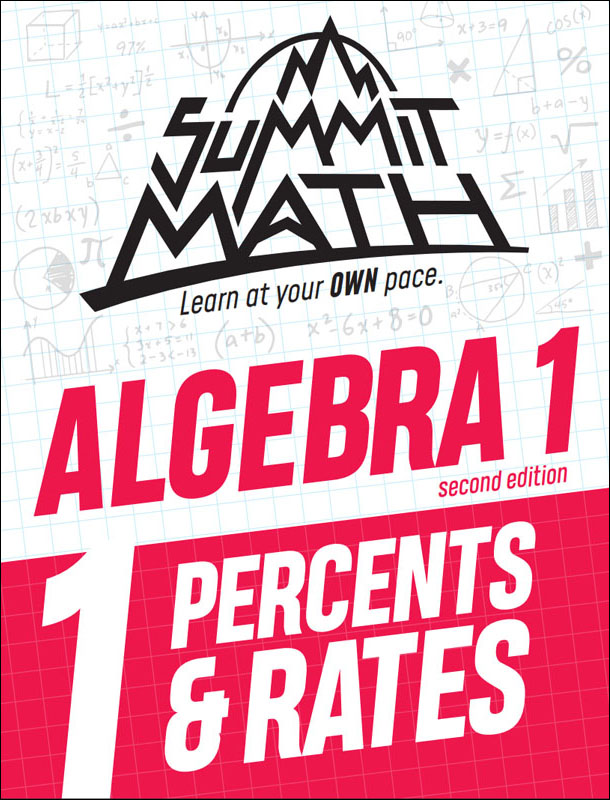•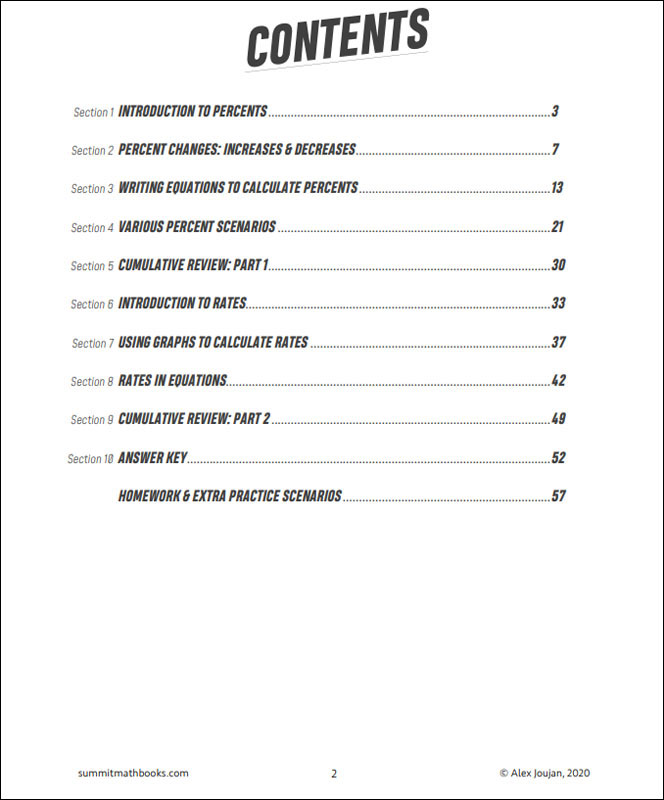•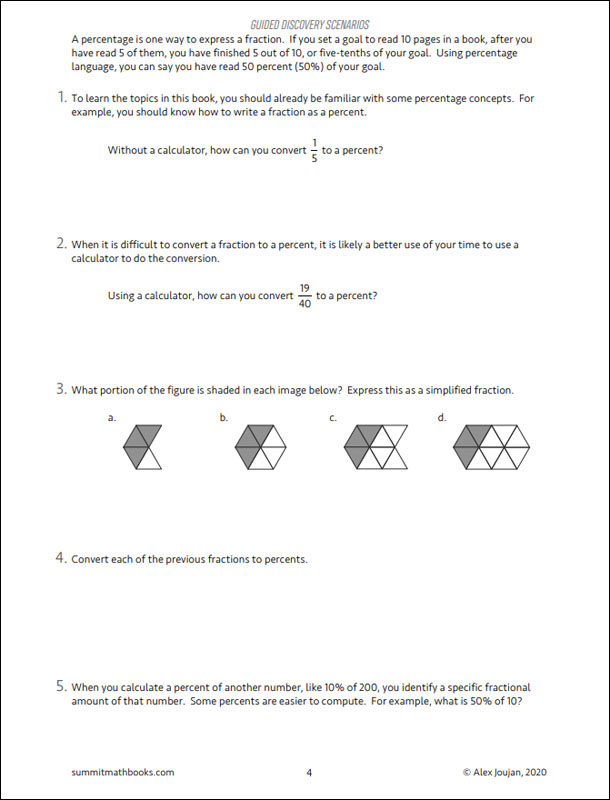•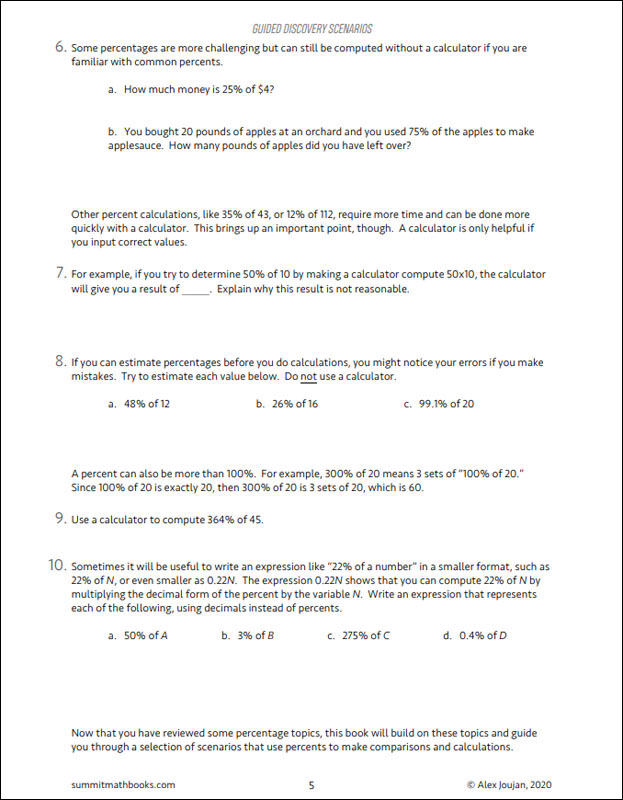•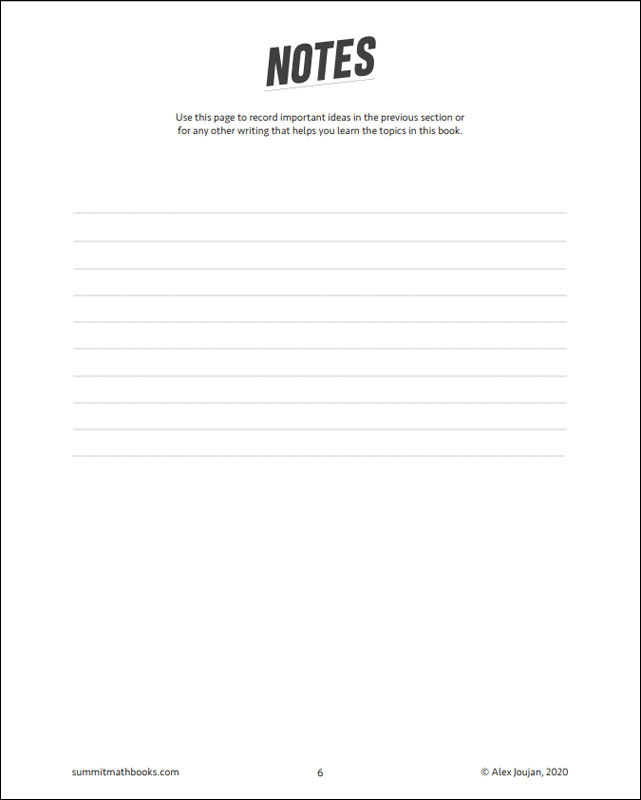•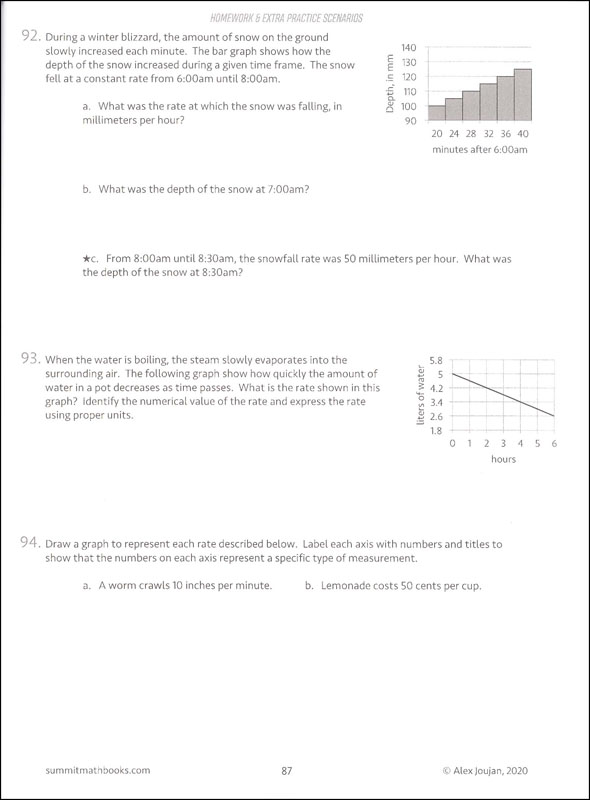# Summit Math Algebra 1 Book 1: Percents & Rates (2nd Edition)

# 078913

Our Price: \$12.25
Retail: \$12.50
Save: 2.00% (\$0.25)
In Stock.
Qty:
Qty:

Item #: 078913 9781713295587 6-10

#### Publisher Description:

This book guides students through a wide variety of percent scenarios. They review percentages as a way to express fractions and then they learn how to calculate percent increases and decreases. Students also learn how to write and solve percent equations that model percent relationships. Percent scenarios come in many forms, so this book shows students a wide variety of percent scenarios. Students learn about rates at the end of the book. They learn how rates can be interpreted from graphs and how rates can be expressed in equations. These rates scenarios are designed to prepare them for learning about linear equations in Algebra 1: Book 2.

Topics in this book:

• Introduction to percents
• Percent changes: increases and decreases
• Writing equations to calculate percents
• Various percent scenarios
• Cumulative review: part 1
• Introduction to rates
• Using graphs to calculate rates
• Rates in equations
• Cumulative review: part 2

Category Description for Summit Math Algebra:

If you are searching for an independent algebra program or a supplement to teach specific algebra concepts, Summit Math is worth your consideration. Using the guided discovery method, students make their own connections as they learn to master each scenario before moving on to the next. Each section builds on previous concepts already learned. The first half of the book incorporates the guided discovery approach, while the latter half uses extra practice sheets to improve understanding of the topics. Each section has an answer key. A placement test and pacing guide is provided for each level. The author states this is a complete program teaching typical topics in the Algebra I and II sequence while preparing students for standardized testing. If students feel they need extra instruction, a video subscription is available from the publisher. Free downloadable tests and a more detailed version of the answer key are also available at the publisher’s website.

Primary Subject
Mathematics
6
10
ISBN
9781713295587
Format
Paperback
Brand Name
Summit Math
Weight
0.7 (lbs.)
Dimensions
11.0" x 8.5" x 0.25"